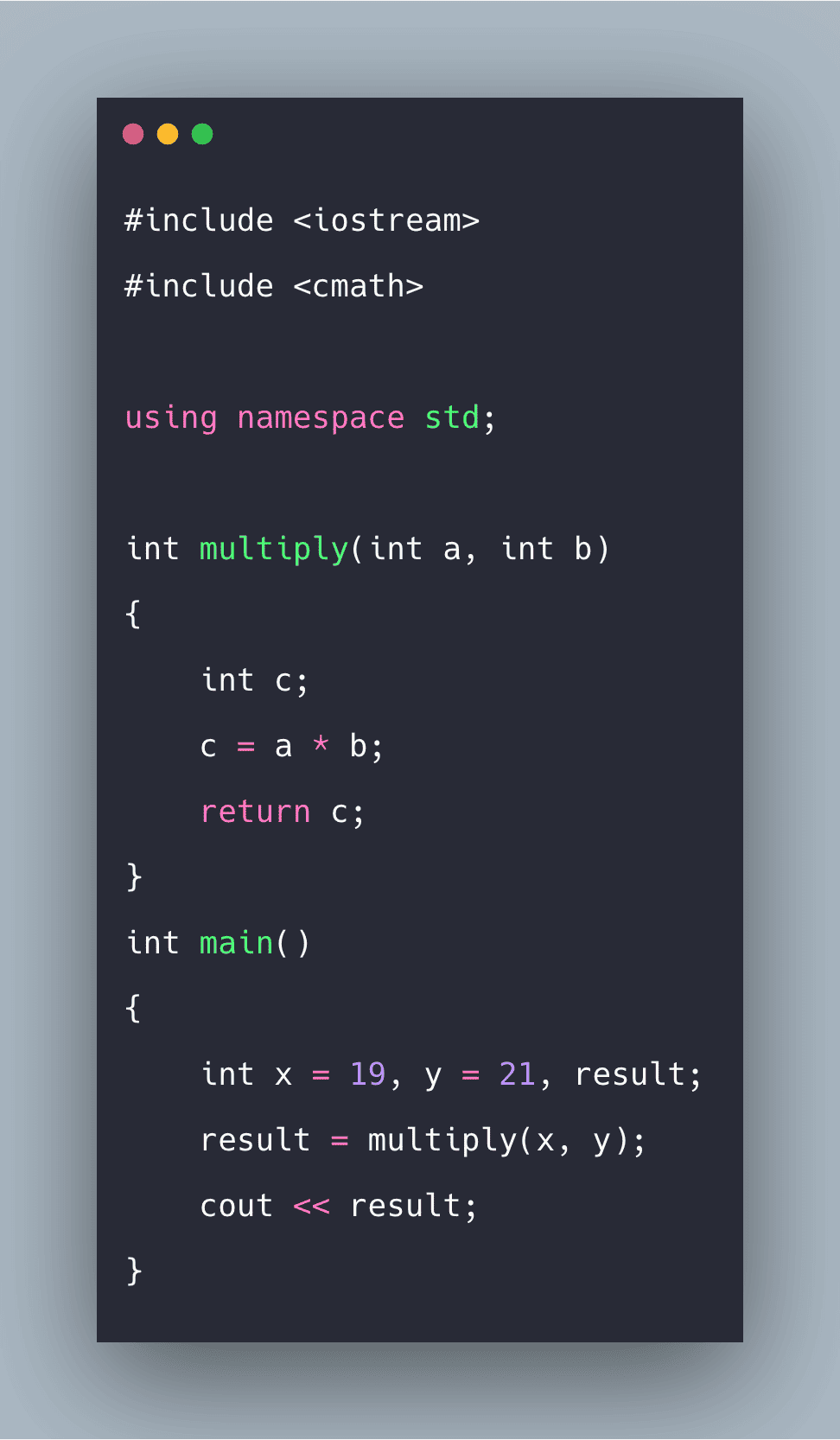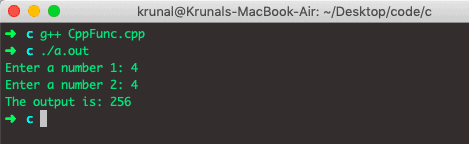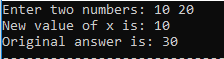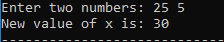# C++ Function: The Complete Guide

0
80Different programming languages name them differently, for example, functions, methods, subroutines, procedures, etc. If you come across any such terminology, then imagine the same concept.

Functions provide better modularity for our application and a high degree of code reusability. You have already seen various functions like printf() and main().

## Why do we need functions?

Suppose in a program there is a task that is performed at several places. So, with different values, we are delivering the same task. This makes our code look lengthy and naïve.

Functions come to the rescue where we can define the task to be performed at one place, pass parameters as required, and get a similar result. Functions make our code modular and more professional.

## C++ Function

C++ function is a group of statements that performs a specific task. A function is the block of organized, reusable code that can be used to perform a single, related action. The good thing about the functions is that they are famous with many names.

There is always a default function in C++ that is main(). Besides, there are two types of functions in c++; they are

1. Built-in function
2. User-defined function

### Built-in function

Built-in functions are already defined in our GCC compiler, and we need to call them with the required parameter. For example: under <string.h> we have many functions, like

###### strlen(string_name): It returns an integer with the length of the string.

Also, there are many built-in functions like, max(), min(), pow(), sqrt() etc.

#### Library Function

See the following example of the Library function.

```#include <iostream>
#include <cmath>

using namespace std;

int main()
{
double number1, number2;
int op;
cout << "Enter a number 1: ";
cin >> number1;
cout << "Enter a number 2: ";
cin >> number2;
op = pow(number1, number2);
cout << "The output is: " << op;
return 0;
}```

See the below output.In the example above, the pow() library function is invoked to calculate the power of a number.

Notice code #include <cmath> in the above program. Here, cmath is a header file. The function definition of pow()(body of that function) is present in the cmath header file.

You can use all functions defined in the cmath library when you include the content of file cmath in this program using #include <cmath> code.

Every valid C++ program has at least one function, that is, main() function.

Now, below, we will discuss the user-defined function.

A user-defined function is a function that is defined by the program according to its needs.

The rule, parameter everything, is written by him.

### Format of function in C++:

``` <return_type>   Function_Name(Parameter 1, Parameter 2,…, Parameter N) {
// Function body
// do stuff with parameters
}
```

The <return_type>  can be int, float, double, long, long int, long double, void, string, and various possible data types.

Function_name follows the same naming convention like it should start with ‘_’ or alphabet followed by a number, alphabet, or ‘_.’

Parameters can be int x, float y, string z, etc.

An example function that calculates the area of the rectangle.

```#include <iostream>
using namespace std;

float areaOfRectangle( float length, float breadth)
{
// Function body
return calculatedArea;
}
int main()
{
// Sample program to show function in C++
// Suppose we have to calculate the area of a rectangle at several places so instead we make a function.

cout << "Enter length and breadth :\n";
// store the result in answer
cout << "Area : " << answer << "\n";
return 0;
}
```

Calling a function is not such a big deal. One has to pass the parameters and call the function by Function_name. It can be seen in the above code where the function areaOfRectangle is called with parameters length and breadth.

## How to Declare Function in C++

To declare a function in C++, use the function prototype. A function prototype is the declaration of a function without its body to give the compiler information about the user-defined function.

The compiler will show the error if the user-defined function is defined after the main() function. It is because the compiler is unaware of user-defined function, types of an argument passed to a function, and return type.

The function prototype in the above example is the following.

`int add(int, int);`

You can see that there is nobody of function in the prototype. Also, there are only return the type of arguments but no arguments. You can also declare a function prototype as below, but writing arguments is unnecessary.

`int add(int a, int b);`

## How to call a function in C++

To call a function in C++, pass the required parameters along with the function name, and if the function returns a value, then you can store returned value.

If we want to execute the codes of the function body, a user-defined function needs to be invoked(called).

In the above program, add(a, b) inside the main() function calls the user-defined function.

The function returns an integer which is stored in the variable.

## How to pass arguments of the function in C++

To pass arguments to a function in C++, it must declare variables that accept the values of the arguments. In the programming language, the argument (parameter) refers to data passed to the function (function definition) while calling it.

In the below example, two variables,  x, and y, are passed to function during the function call. These arguments are known as actual arguments.

The value of a and b are initialized, respectively. These arguments a and b are called formal arguments.

Inside the main function, we call the multiply() function, in which we pass the actual parameters x and y.

So, in the below code int a and int b are formal parameters, and int x and int y are actual parameters.

See the following code.

```#include <iostream>
#include <cmath>

using namespace std;

int multiply(int a, int b)
{
int c;
c = a * b;
return c;
}
int main()
{
int x = 19, y = 21, result;
result = multiply(x, y);
cout << result;
}```

Talking about parameters, parameters can be passed in two ways, namely:

1. Call by Value
2. Call by reference

### Using Call by Value

In this method, just the values of the parameters assigned are passed, so any changes made to the parameters passed inside the function body will not change their value in the main function.

```#include <iostream>
using namespace std;

int dostuff( int x, int y)
{
// Function body
x = x+y; // change made here will not be reflected in main.
return x;
}
int main()
{
// Sample program to show call by value.
int x,y;
cin >> x >> y;
int answer = dostuff(x,y); // Pass the parameters
// Value of x will not be changed here.
cout << x;

return 0;
}
```

In the code above, x and y have been passed by value to dostuff. In the function dostuff, x has been changed to x+y, but this change is not reflected in the main function.### Using Call by Reference

In this method, parameters passed into the function are referenced with arguments of the function using the operator ‘&’. Changes made to reference variables inside the body function will be reflected inside the main function.

```#include <iostream>
using namespace std;

int dostuff( int &x, int y)
{
// Function body
// Look for '&' before x in int &x above.
x = x+y; // change made here will be reflected in main.
return x;
}
int main()
{
// Sample program to show call by reference.
int x,y;
cin >> x >> y;
int answer = dostuff(x,y); // Pass the parameters
// Value of x will be changed to x+y here.
cout << x;

return 0;
}
```

The above code tells about the call by reference type passing of parameters. The x parameter has been passed as a reference variable. Inside the argument of function dostuff, int &x  is used instead of int x. Changes to x, i.e., x=x+y, are reflected in the main function.#### Can we pass fewer parameters than defined in the function definition?

Yeah. This can be done using default parameters. Default parameters should be present only at the end of the arguments.

The below example will explain the concept.

```#include <iostream>
using namespace std;

int multiply( int x, int y,int z=1)
{
// Function body
// If only two inputs are there then z will be 1.
return x*y*z;
}
int main()
{
// Sample program to show default parameters.
int x,y,z;
x=4; y=10; z=3;
// Multiplication of 3 numbers
cout << multiply(x,y,z) << "\n";
// Multiplication of 2 numbers same function can be used
cout << multiply(x,y) << "\n";

return 0;
}
```

Here the multiply function can be used for both 3 numbers and 2 numbers. z has been set by default to 1. Notice that it has been placed at the end of the arguments. If only two arguments are passed, it will set the value of z=1.

That’s it for this tutorial.

This site uses Akismet to reduce spam. Learn how your comment data is processed.